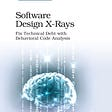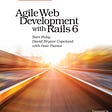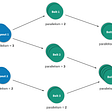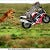# Python’s Pandas dictionary-1 (Basics)

Most of the times we use .csv and .xlsx formats of Excel file, so discuss how to read or import those files here.
2. a. Import CSV file:
`import pandas as pddf = pd.read_csv(r’My_folder\Pandas\file_name.csv’)#[OR] here we use r’ ‘ for having ‘\’df = pd.read_csv(‘My_folder/Pandas/file_name.csv’)`
`import pandas as pd#for importing .xlsx filedf = pd.read_excel(r‘My_folder\Pandas\file_name.xlsx’)#for importing .xls filedf = pd.read_excel(r‘My_folder\Pandas\file_name.xls’) `
`#a. Print top 5 rows of dfdf.head() #[OR]df[:5]#b. Print last 5 rows of dfdf.tail() #[OR]df[5:]`
`df.info()`
`#If all the values are numeric without letters or special characters.df[‘column1’] = df[‘column1’].astype(‘int’)df[‘column1’] = df[‘column1’].astype(‘float’)(or)df[‘column1’] = pd.to_numeric(df[‘column1’])`
`df[‘column1’] = df[‘column1’].astype(‘object’)`
`df[‘column1’] = pd.to_datetime(df[‘Date’])`
`#To check mean (Average) of all numeric columns.df.mean()#To check mean of column.df[‘Column_name’].mean()`
`#To check Median of all numeric columns.df.median()#To check Median of column.df[‘Column_name’].median()`
`#To check Mode of all numeric columns.df.mode()#To check Mode of column.df[‘Column_name’].mode()`
`#For all columnsdf.describe()#For selected columns.df[[‘Column1’, ’Column2’, ‘Column3’]].describe()`
`#Drop columns:df.drop([‘Column1’,’column2’], axis=1)#Drop rows from index 1:10df.drop(df.index[1:10])#Or if you want to drop rows based on condition or a column categorydf.loc[df['column1'] != 'Blank'] #ORdf.loc[~df['column1'] == 'Blank']`
`df.isnull().sum()`
`df.isnull().sum()/len(df.index)`

--

--

--

## More from Maniswaroop

Love podcasts or audiobooks? Learn on the go with our new app.

## What’s LDV and Why Does it Matter for My Nonprofit or School? Part II## Ansible: Introduction and case studies## Top 6 Challenges in Software Migration for Startup## Introduction to Automated Testing using Selenium - GSoC ’18 Week 7## Organizing Structures## Creating Machines## Introduction to Apache Heron## Python Set Methods## R vs MatLab: The Key Difference Between Two Programming Languages## Pandas